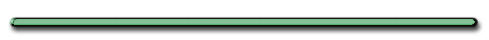Frame of Reference

Producer: Richard Leacock Production Company: Educational Development Corp. Sponsor:
Eric Prestamon Audio/Visual: sound, black and white PSSC

Motion in a Plane

Plane - 2 dimensional surface

What kinds of objects have
2 dimensional motion?

Objects Moving
Along the Earth's Surface

Planets around sun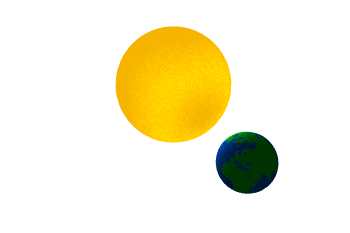PendulumsProjectilesHuman Cannonball
at the 2007 State Fair of Texas

Projectile Fired Horizontally

Review

Object projected horizontally1. Viy = ?

 Viy = 0

2. ay = ?

 ay = 9.8 m/s2

3. Horizontal Velocity?

(no air friction)

 Horizontal Velocity is Constant (no air friction)

Vix = Vx = Constant

Horizontal Distance Traveled

 dx = Vxt (Memorize)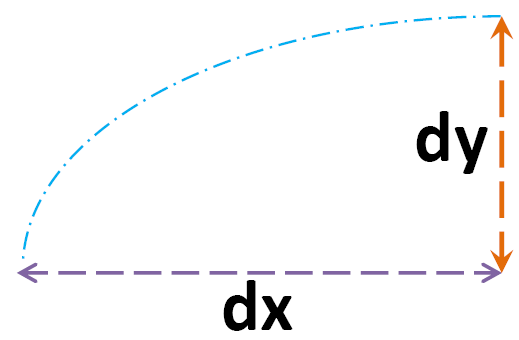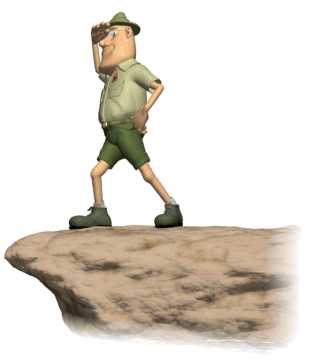4 objects thrown horizontally,

same height,

at various speeds.

Compare their:

 Viy? Same (Viy = 0) ay? Same ay = 9.8 m/s2 Vy? Same for all height (dy)? Same at all times time spent in air same

 Ranking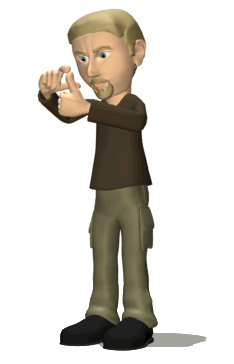Task All objects below are thrown horizontally. Rank the time each spends in the air from longest to shortest.June 1997 #64

Object is thrown horizontally,
only height
determines the time in air.

 Ex) A baseball is thrown horizontally from a grandstand 20 m above ground at speed of 10 m/s. (a) How long will the ball remain in flight before reaching ground?

Ex) A baseball is thrown horizontally from a grandstand 20 m above ground at speed of 10 m/s.

(a) How long will the ball remain in flight before reaching ground?

 Horizontal Info Vertical Info Vx = 10 m/s Viy = 0 (thrown horizontally) ay = +9.8 m/s2 dy = 20 m t = ?

Step 3 - Substitute and Solvedy = Viyt + ½ayt2

20 m = 0 + ½(9.8 m/s2)t2

t = 2 seconds

(b) What is the projectile's maximum range before it hits ground?

(b) What is the projectile's maximum range before it hits ground?

Range?Range (dx)

 Horizontal Vertical Vx = 10 m/s Viy = 0 (thrown horizontally) dx = ? ay = +9.8 m/s2 t = 2 sec dy = 20 m t = 2 sec

Range

Horizontal Displacement (dx)

dx =Vxt

= (10m/s)2 secs

= 20 m

| Dartfish |

| Onsite |

Review

Which velocity is constant for all spheres?.

Vx

AP Question

Hewitt Question

A cannonball is fired horizontally at 10 m/s from a cliff. Its speed one second after being fired is about

A. 10 m/s.
B. 14 m/s.
C. 16 m/s.
D. 20 m/s.

Vx = Vy = 10 m/s

V = 14 m/s

AP Physics

Review of freefall

Ex) Compare the time it takes for a whiffle ball to go up with the time it takes to reach its initial position.

Takes longer to return from peak. Friction would cause avg. vel.  to be less on the way down.

Ex) A baseball is dropped from the top of a bleachers from a height of 80.‑meters.  At the same time, another ball is thrown from horizontally.

Both balls hit level ground, 50. m apart.  (Air resistance is negligible.)

Find the horizontal velocity of the ball thrown.

Vx = dx/t

Time in air

∆dy = Viyt + 1/2ayt2

∆dy = 1/2ayt2

80 m = (4.9 m/s2)t2

t = 4 sec

Vx = dx/t

Vx = dx/t  = 50 m/4 sec

= 13 m/s

Ex) An object moving horizontally with speed V falls off the edge of a vertical cliff and lands a distance d from the base of the cliff.

How far from the base of the cliff would the object land if the height of the cliff was doubled?

dx = Vxt = d

dy = 1/2at2

double dy then t increased by radical 2

Ex) From a cliff of height 28.8 m above the ground, a ball is thrown horizontally with an initial velocity of 7.0 m/s.

What is the range of the ball?

dx = Vxt

∆dy = 1/2ayt2

28.8 m = 4.9t2

t = 2.4 sec

dx = Vxt

= 7.0 m/s(2.4 s)

dx =  17 m